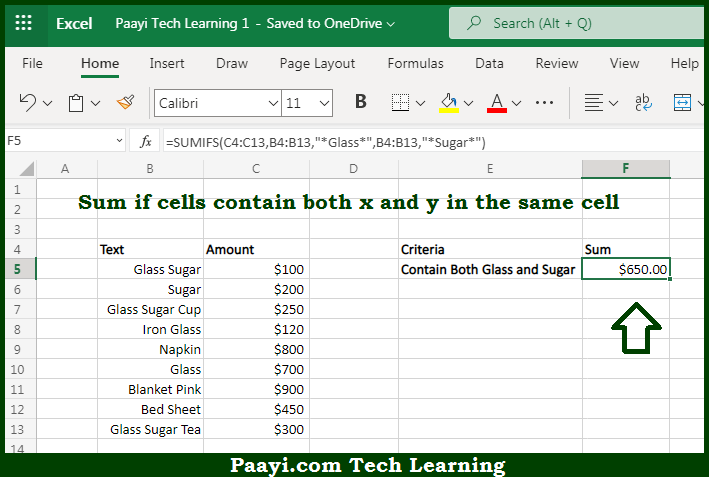# Learn How to SUM If Cells Contain Both x and y in Microsoft Excel

Written by | 0 Comments | 462 Views

In this article, you will learn how to SUM various things in Microsoft Excel using a single/combination(s) of functions. You will also know how to SUM If Cells Contain Both x and y and see the generic formula.

SUM If Cells Contain Both x and y in Microsoft Excel

The main purpose of this formula is to sum if cells contain both x and y. Here we will learn how to sum if both the cell is having x and y in the workbook in Microsoft Excel. That implies, with the help of a formula based on the SUMIFs function you can able to sum if cells contain both x and y. So, with the help of this formula, you can able to sum if both the cell is having x and y in the workbook in Microsoft Excel.

General Formula to SUM If Cells Contain Both x and y

=SUMIFS(rng1,rng2,"*cat*",rng2,"*rat*")

The Explanation for the SUM If Cells Contain Both x and ySo we know that with the help of the given formula above you can able to sum if cells contain both x and y. Here we will learn how to sum if both the cell is having x and y in the workbook in Microsoft Excel. As we know that the SUMIFS function is based on AND logic it's having an automatic behavior. We have to supply two range/criteria pairs, both operating on the same range. For both criteria, we are using an asterisk, which is a wildcard that matches "one or more characters". We put an asterisk at the start and end to allow the formula to match in both the cells. When both criteria return TRUE in the same row, SUMIFS sums the value in the targeted column. So, with the help of this formula, you can able to sum if both the cell is having x and y in the workbook in Microsoft Excel.